﻿ 舰船显控台动力学仿真分析研究
 舰船科学技术2023, Vol. 45Issue (1): 163-166    DOI: 10.3404/j.issn.1672-7649.2023.01.029PDF

Research on dynamic simulation analysis of display console
ZHAO Jin-peng
Jiangsu Automation Research Institute, Lianyungang 222006, China
Abstract: This paper takes a certain type of ship display console as the research object. In order to solve the overall structural design strength of the display console to meet the requirements of the environmental adaptability impact test of electronic equipment. An Ansys finite element analysis was carried out on the three-dimensional structural design model of the display console. First, the corresponding finite element model is created, and the impact constraints under the actual working conditions are imposed. Then, according to the finite element model, the transient dynamic simulation analysis and harmonious response simulation analysis are gradually carried out. Finally, the stress and displacement of the display console under the impact load and external excitation are obtained, and the structural optimization is carried out for the parts with large stress and displacement after the display console is subjected to the impact load and external excitation, which meets the environmental adaptability impact of electronic equipment.
Key words: display console     finite element     transient dynamics     harmonic response     simulation analysis
0 引　言

1 创建显控台有限元模型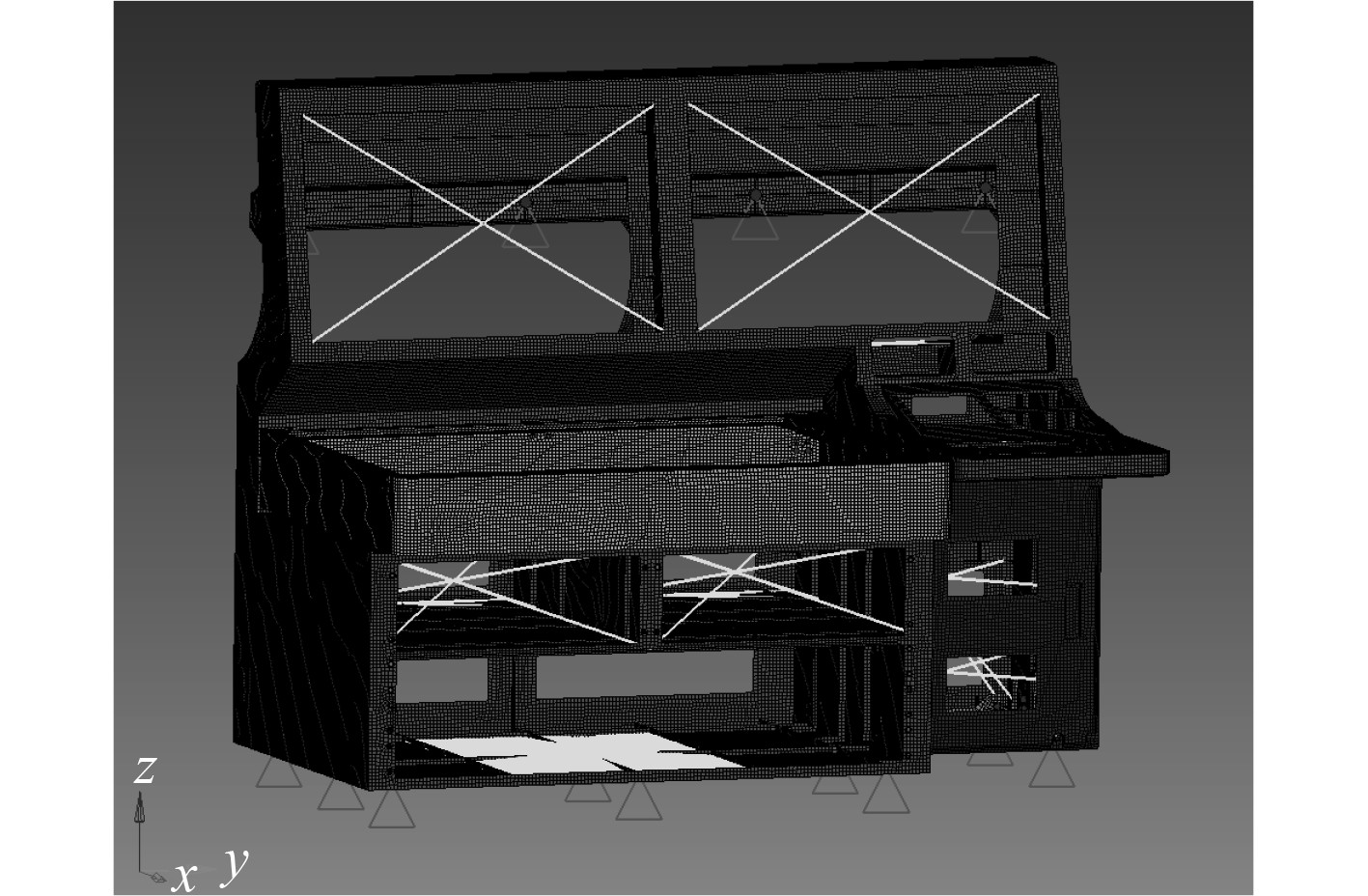图 1 显控台有限元模型 Fig. 1 Finite element model of display console

2 瞬态动力学分析理论

 ${\boldsymbol{ M}}{\ddot {u}} + {\boldsymbol{B}}{\dot {u}} + {\boldsymbol{K}}u = P(t) ，$ (1)
 $u(t = 0) = {v_0}。$ (2)

 $u = Xd ，$ (3)

 ${X^{\rm{T}}}{\boldsymbol{M}}X\ddot d + {X^{\rm{T}}}{\boldsymbol{K}}Xd = {X^{\rm{T}}}P ，$ (4)

 ${X^{\rm{T}}}{\boldsymbol{M}}X\ddot d + {X^{\rm{T}}}BXd + {X^{\rm{T}}}{\boldsymbol{K}}X = {X^{\rm{T}}}P ，$ (5)

 ${m_i}{\ddot d_i}(t) + {b_i}{\dot d_i}(t) + {k_i}{d_i}(t) = {p_i}(t)，$ (6)

 ${\ddot d_i}(t) + 2{\varsigma _i}{\omega _i}{\dot d_i}(t) = \frac{1}{{{m_i}}}{p_i}(t) 。$ (7)

 $\tilde P(\varOmega ) = \int_{t = 0}^0 {P(t)} {e^{ - i\varOmega t}}{\rm{d}}t ，$ (8)

 $\tilde u(\varOmega ) = h(\varOmega )\tilde P(\varOmega ) ，$ (9)

 ${u_t} = \frac{1}{\text{π} }\int_{\varOmega = 0}^\infty {{Re} \left[ {u(\varOmega ){e^{i\varOmega t}}} \right]{\rm{d}} \varOmega } 。$ (10)
3 瞬态动力学仿真分析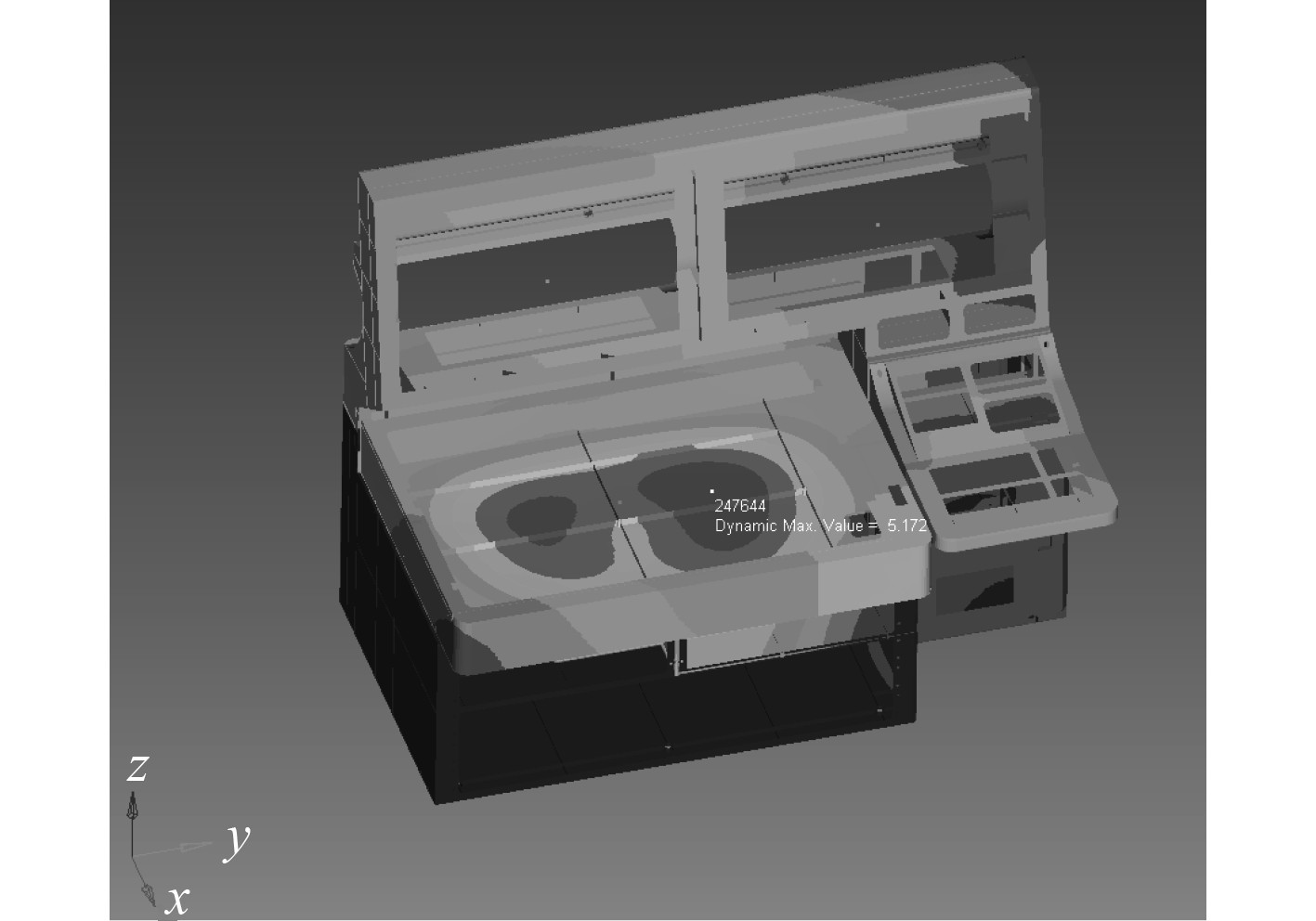图 2 显控台位移最大点位置示意图 Fig. 2 The schematic diagram of the display console's maximum displacement point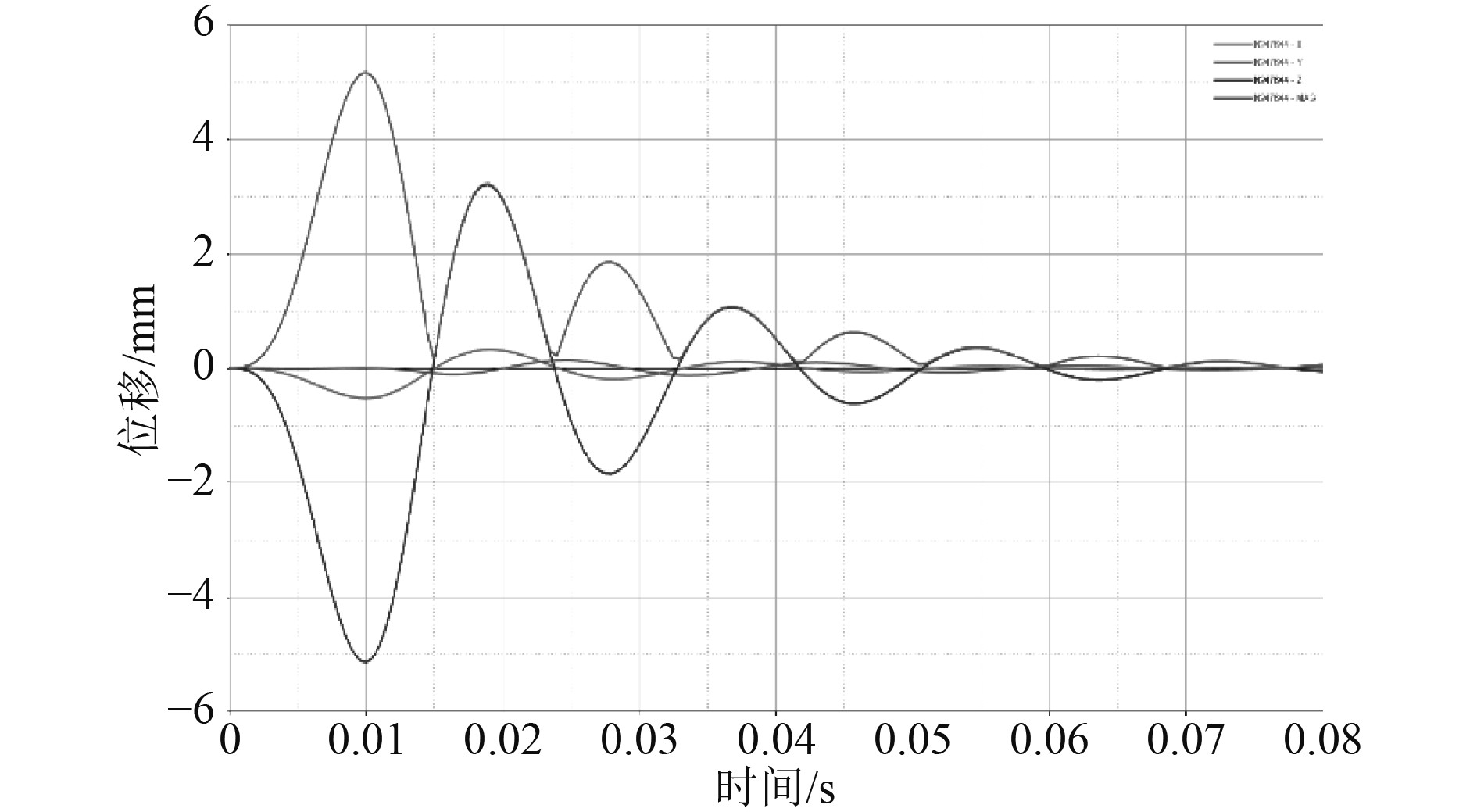图 3 显控台最大位移点时间-位移曲线 Fig. 3 The time-displacement curve of the display console's maximum displacement point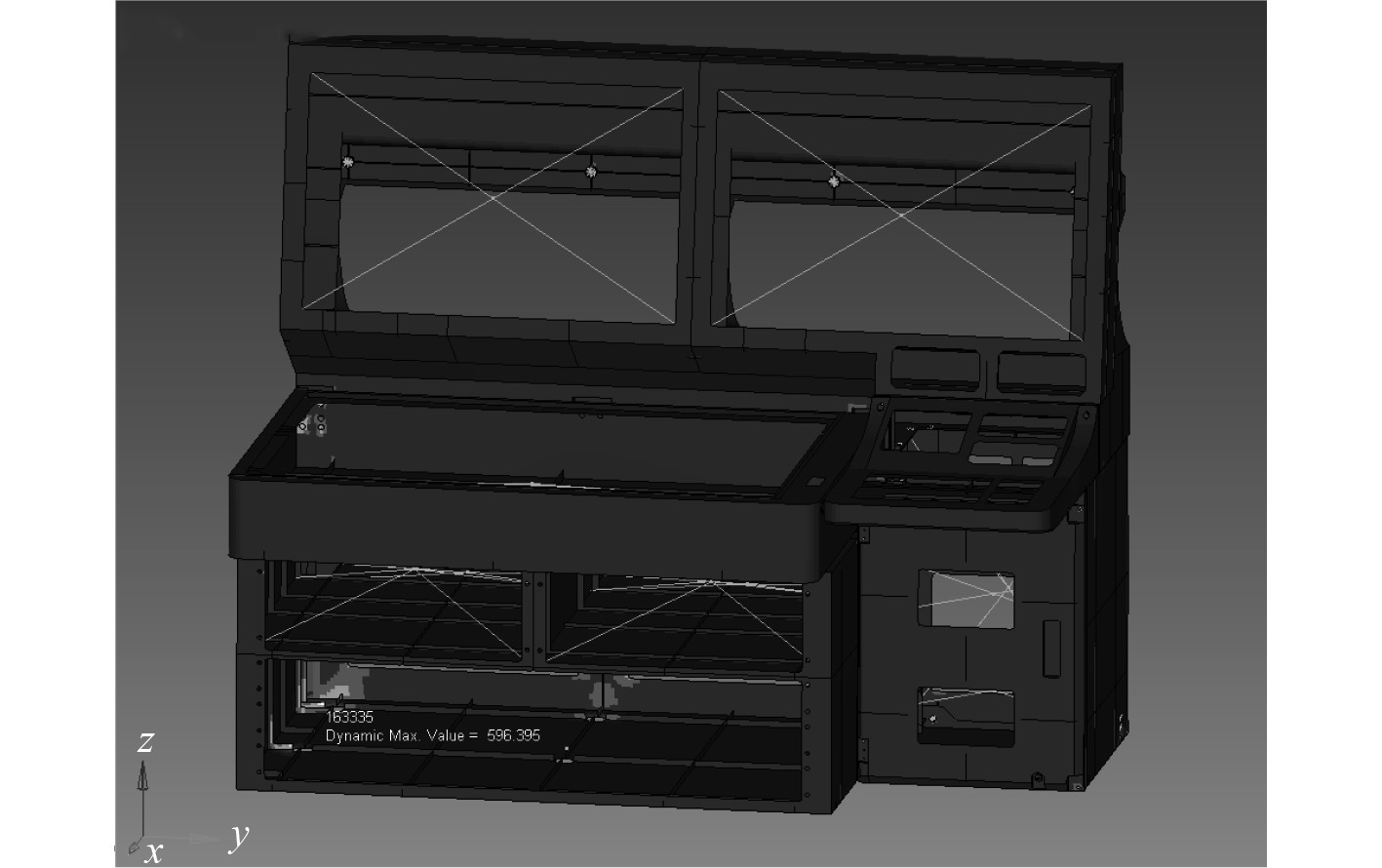图 4 显控台最大应力点位置示意图 Fig. 4 The schematic diagram of the display console's maximum stress point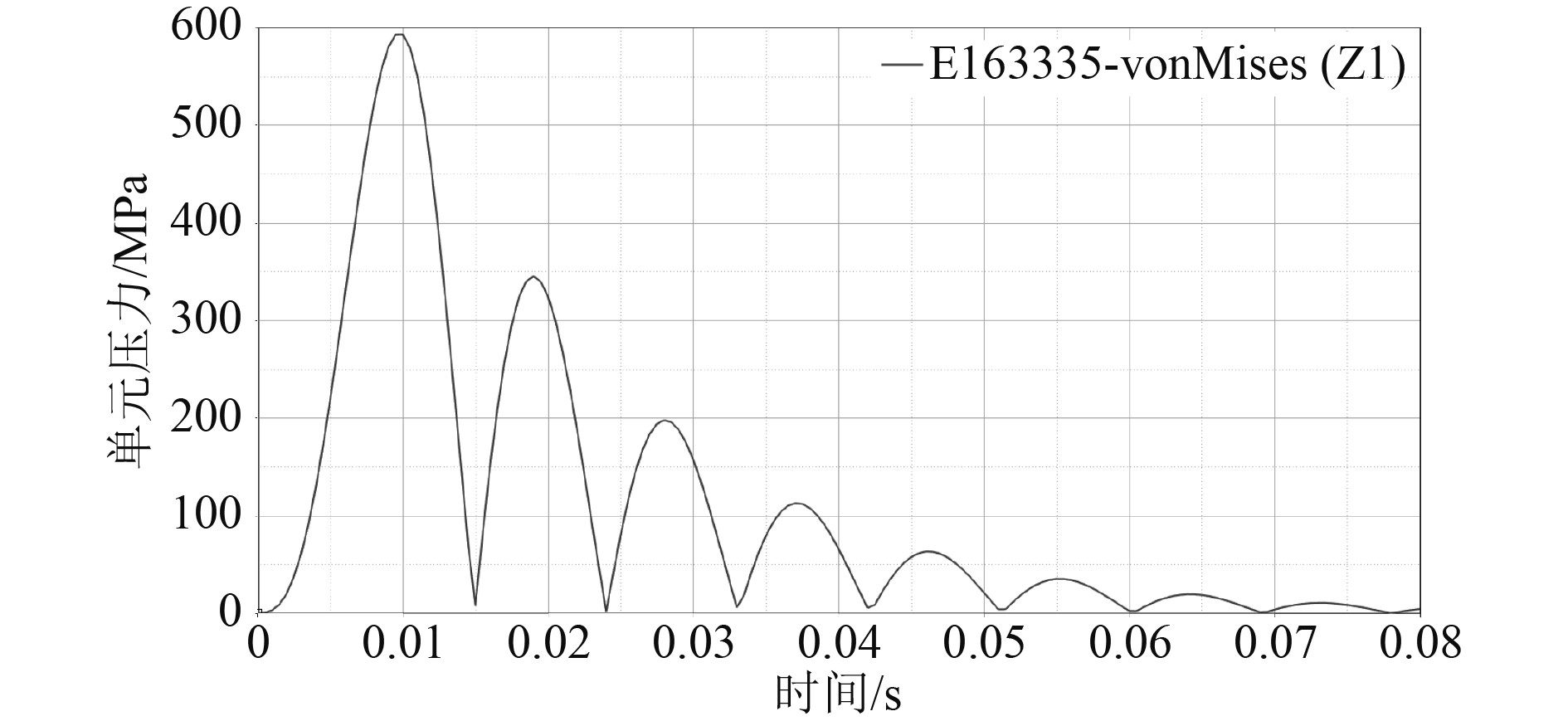图 5 显控台最大应力点应力-时间曲线 Fig. 5 The stress-time curve of the display console's maximum stress point
4 谐响应分析理论

 ${\boldsymbol{M}}\ddot u + {\boldsymbol{B}}\dot u + {\boldsymbol{K}}u = P{e^{i\varOmega t}}，$ (11)

 $u = d{e^{i\varOmega t}} ，$ (12)

 $(K - {\varOmega ^2}M + i\varOmega B)d{e^{i\varOmega t}} = P{e^{i\varOmega t}} 。$ (13)

 $u = X{d^{^{i\varOmega t}}}，$ (14)

 $( - {\varOmega ^2}{m_i} + i\varOmega {b_i} + {k_i})d{e^{i\varOmega t}} = {p_i}{e^{i\varOmega t}}，$ (15)

 $( - {\varOmega ^2}{m_i} + i\varOmega {b_i} + {k_i}){d_i} = {p_i}。$ (16)
5 谐响应仿真分析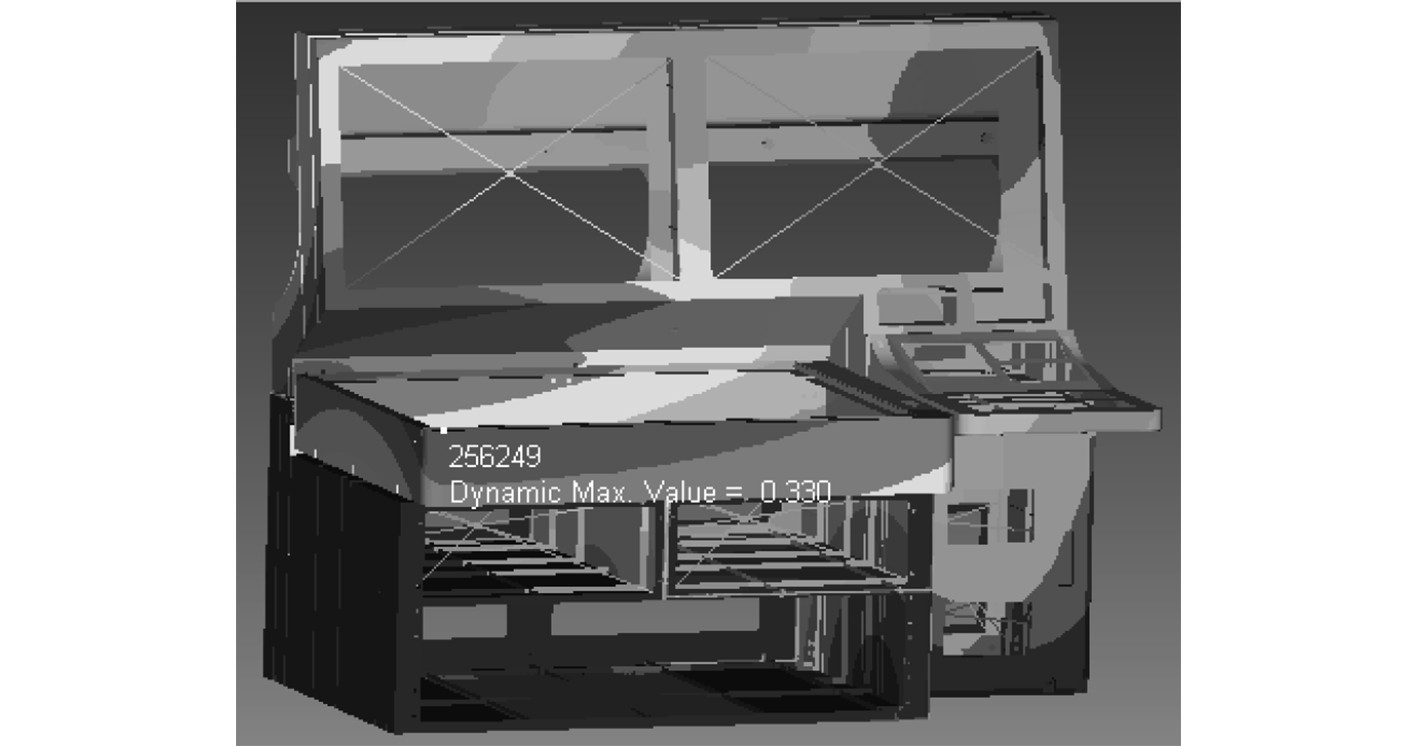图 6 x方向激励海图谐响应最大位移点 Fig. 6 x direction excited chart harmonic response maximum displacement point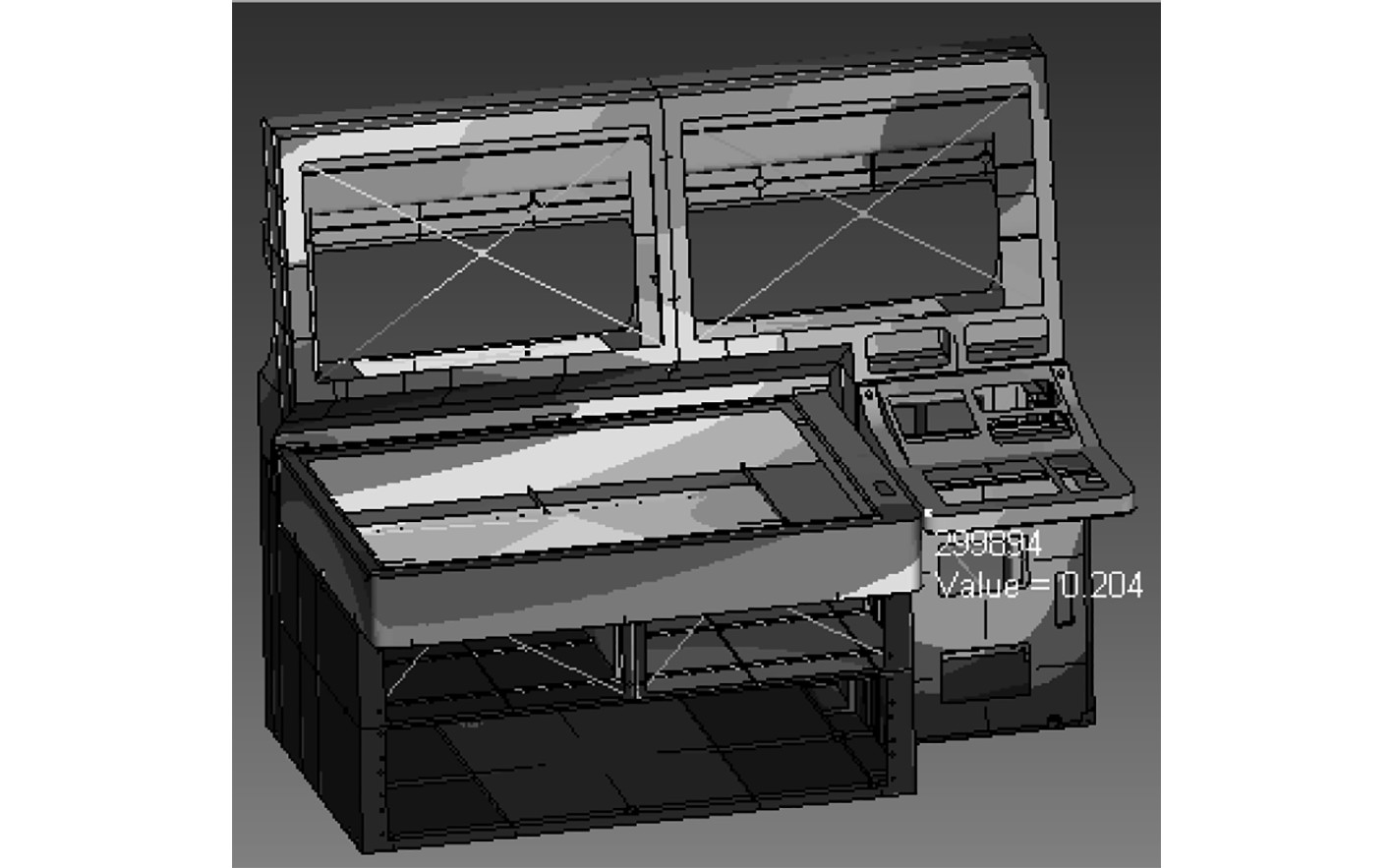图 7 x方向激励操作台谐响应最大位移点 Fig. 7 x direction excitation platform harmonic response maximum displacement point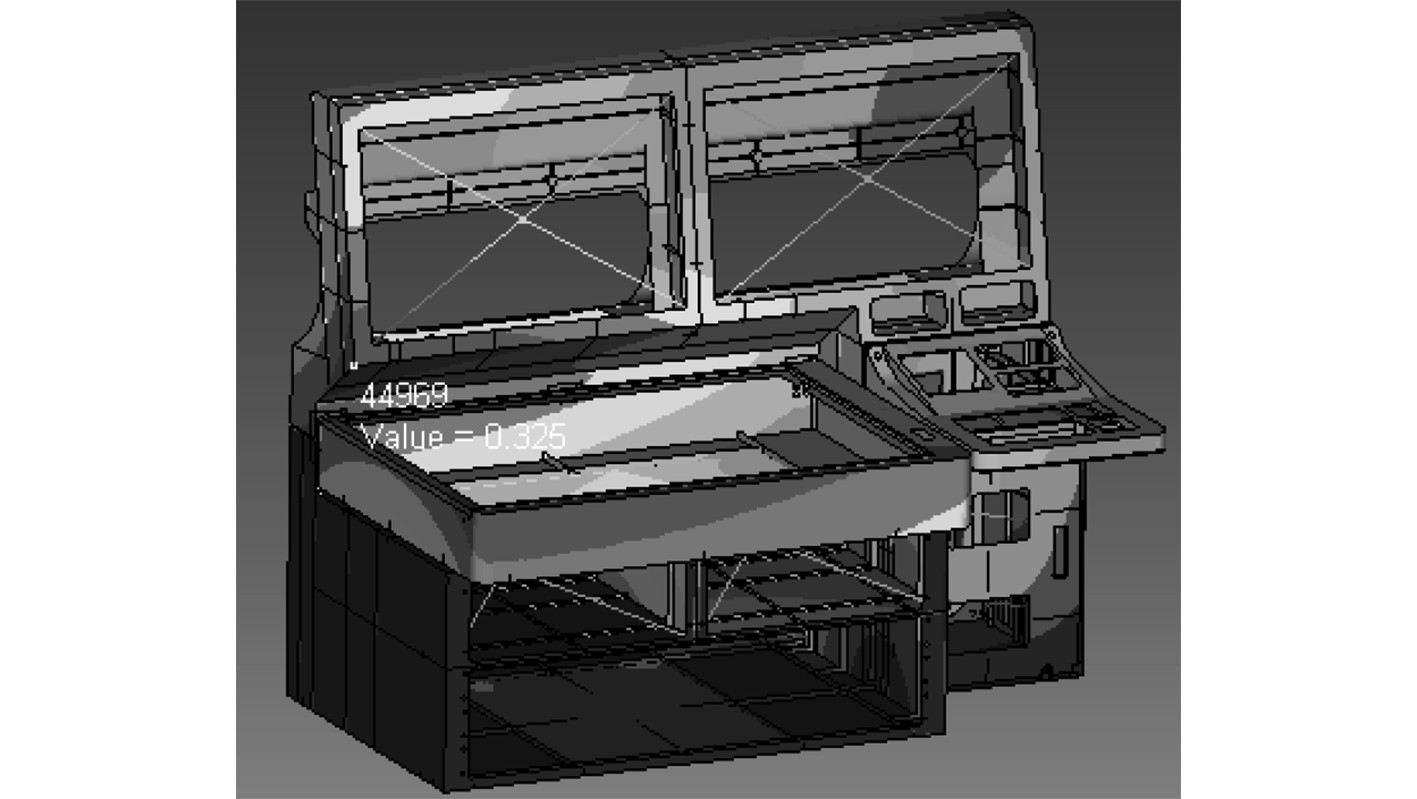图 8 x方向激励显示器谐响应最大位移点 Fig. 8 x direction excitation display harmonic response maximum displacement point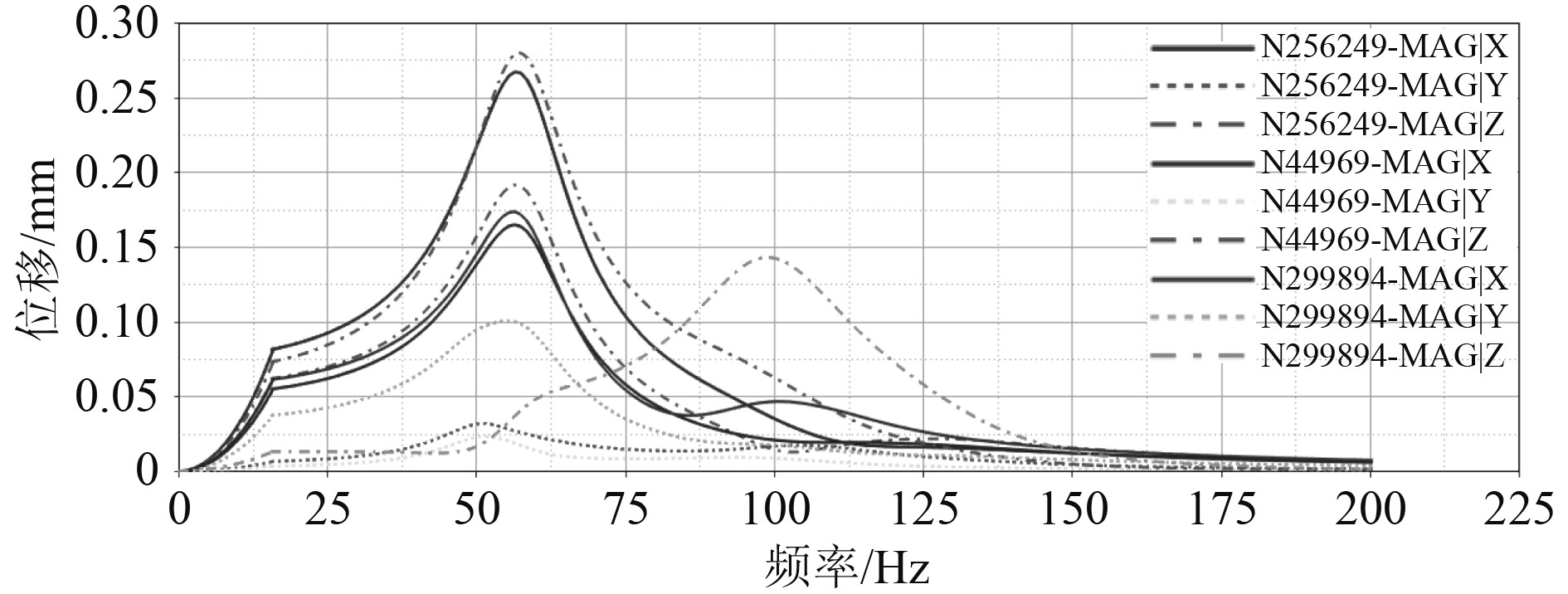图 9 x方向谐响应分析最大位移点位移-频率曲线 Fig. 9 x direction harmonic response maximum displacement-frequency curve

6 结　语

1）显控台在冲击载荷作用下，最大位移为5.17 mm，最大应力接近600 MPa，瞬态的最大应力超过显控台本身材料（铸铝ZL201）的许用应力335 MPa，会损伤显控台本身结构。

2）显控台在x方向外部激励作用下，海图部件的谐响应位移最大，最大位移为0.329 mm，最大位移对应的频率为56.5 Hz。

3）显控台在x方向外部激励作用下，显示器沿z轴方向位移最为突出；操作台在56.5 Hz时延x轴方向位移最大，在99.5 Hz时延z轴方向位移最大。

4）在舰船显控台设计初期，就要加强海图、显示器和操作台等部件的强度和刚度设计，结合仿真分析，对底部11个减振器和背部4个减振器进行优化选型，避免舰船显控台受冲击载荷和外部激励作用带来的损伤和破坏。

  赵金鹏. 舰船显控台噪声分析试验研究[J].舰船科学技术. 2019, 41(9): 134−136.  孙帆. 机载碳纤维显控台的设计研究[D]南京: 南京理工大学. 2013.  周海安. 基于工程应用的加肋板结构的振动特性分析[J]. 舰船科学技术. 2019, 41(9): 42−47.  谭亮. 船载情报指挥控制台的系统设计[C]// 2015年CAD/CAM学术交流会议论文集. 2015, 177−184.  梅真, 郭子雄. 磁流变阻尼减振结构振动台试验与动力可靠性分析[J]. 湖南大学学报(自然科学版). 2017, 44(7): 41−48.  严宏. 基于拓扑优化的某机载显控台轻量化设计[J]. 机械与电子, 2016, 34(11): 22−25.  杨玉光, 周吉, 李齐兵. 某机载显控台结构的有限元分析[J]. 电子机械工程. 2016, 32(4): 62−64.  孙帆. 某碳纤维显控台结构动力学仿真分析及优化[J]. 电子机械工程. 2015, 31(4): 57−59.  梅真, 侯炜, 郭子雄. 黏滞阻尼减震结构振动台试验与动力可靠度分析[J]. 振动与冲击. 2018, 37(3): 136−142.  航燚, 孙帆, 沈文军. 直升机载显控台设计与试验[J]. 电子机械工程. 2015, 31(1): 39−45.